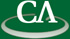## Prime Decomposition of Ideals in Polynomial Rings

#### Stephen Melczer, Department of Mathematics, Simon Fraser University.

Wednesday June 23rd in K9509 at 3:30pm.

```Abstract.

Given an ideal I in a polynomial ring, two fundamental questions that arise in
algebraic geometry are to determine the radical of I and to represent the radical
as the intersection of prime ideals.  In the case where I has Hilbert Dimension
0 there are well known and efficient algorithms for solving these problems.  We
will give an overview of these algorithms and discuss how, through a suitable
decomposition, they can be used in the case of positive dimensional ideals.
Finally, we discuss how pre-processing a positive dimensional ideal using certain
splitting techniques can result in a large increase in performance.  Results
comparing these algorithms with and without pre-processing in Maple will be shown.
```